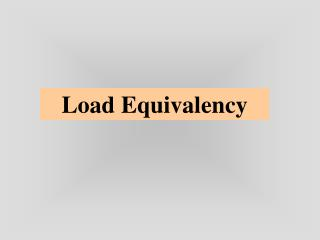DownloadDownload PresentationDownload Presentation- - - - - - - - - - - - - - - - - - - - - - - - - - - E N D - - - - - - - - - - - - - - - - - - - - - - - - - - -
##### Presentation Transcript

2. Load Equivalency Plate theory: solutions limited to a single tire print Prickett & Ray (1951) – provided a graphical extension of Westergaard theory to multiple-wheel loads (pattened after Newmark’s extension of Boussinesq soln.) - resulted in PCA code “Airport”- int. - dimensionless ratios a=loaded radius, s=tire spacing

3. Kreger (1967) – H51 program - Dense liquid edge stress • Peutz et al (1968) – Burmister linear-elastic theory - BISAR code allowed for multiple-wheel loads • Yoder & Witczak (1975) • Used dimensional analysis in a graphical summary of Pickett & Ray charts d • Edge • Interior • Single, dual, or dual tandem wheel loads L x

4. Asphalt Institute • - used s/a • FAA • based on tire configuration) • Effort has always been to transform the actual multiple-wheel load into an equivalent loading system consisting of a single wheel. • ESWL: Equivalent Single Wheel Load • ESAL: Equivalent Single Axle Load • ESAR: Equivalent Single Axle Radius

5. ESWL COE (1940); CBR Design Method(B-29 Aircraft Loading) Load & wheel spacings combinations that yield a LEF of 1 when compared to any “given” single axle. Boussineq soln • LEF depended on: • wheel spacing and dual spacing • mechanistic response • layer thickness & moduli

6. Equal Vertical Stress (Boyd & Foster - 1950)

7. Maximum Vertical Stress or Deflections

8. Equal Vertical Deflection (Boyd & Ahlvin - 1958) As long as Sd/a and h1/a remain the same, the load factor will be the same. • Dimensional analysis • s/a • r/a • z/a

9. Equal Tensile Strain

10. Equal Contact Pressure

11. Gerrand & Harrison – 1970 • used all responses. • used super positioning • abandoned constant pressure assumption • equated the contact area of the ESWL to that of one wheel of the assembly

12. ESAL • AASHTO (AASHO) Rd Test • ESAL concept developed in 1940 – adopted by the Calif. Div of Highways • AASHO based it on PSI; mainly roughness (but intended originally for fatigue induced distress) Nonetheless, AASHO’s entirely statistical-empirical ESAL concept is based on the assumption that the destructive effect of a number of applications of a given axle group (defined in terms of load magnitude and configuration) can be expressed in terms of a different number of applications of a standard or base load. (Ioannides et al 1993) Linear cumulative damage (Miner’s fatigue hypothesis) - been used for everything from rutting to erosion & pumping.

13. ESAL Approach was less mechanistic; more statistical • Decon (1969): attempted to ‘prove’ the ESAL, i.e. EALF = Wt18/Wtx=(εx/ε18)γ; γ=4 • Suggested using γ = 5.5 • Proof of ESWL concept never done • Irick & Hudson (1964) • Stated 4th power law applied to a ratio of deflections • Scala (1970): LEF based on load induced deflection but is not independent of pavement structure.

14. OECD (1988) • Refuted 4th power law • concluded that not possible to prove the existence of a law of equivalence between loads in terms of their damaging effects on pavements. • the power () is different for each pvmt type. •  = 4 Rutting & Fatigue •  = 11 to 33 for semi-rigid •  is sensitive to infrequent heavy loads •  varies from 1.2 to 8 if the standard load is well chosen • Every country has a different  for the same design !

15. ESAR • The radius of an equivalent single wheel that gives the same response as a dual assembly for the same load. • governed by For interior loading(Tayabji et al 1987)Max bending stress (superposition) (Ioannioes et al 1993)

16. Equate (For equal P)

17. Traffic Analysis • ni = (n0)i(G)(D)(L)365(Y) (n0)i = initial number or reps/day (G) = growth factor (D) = directional distribution factor (L) = lane distribution factor (Y) = design period - yrs

18. Traffic Analysis pi = % of total reps for the ith load group Fi = EALF for the ith load group (ADT)0= initial avg daily truck traffic T = % of trucks A = avg axles per truck Tf = truck factor = # of 18k SAL applications per truck